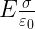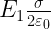Enlightened

# Question 28: NCERT Solutions for 12th Class Physics: Chapter 2-Electrostatic Potential and Capacitance

• 0

Question 28: NCERT Solutions for 12th Class Physics: Chapter 2-Electrostatic Potential and Capacitance

Show that the force on each plate of a parallel plate capacitor has magnitude equal toQE, where Q is the charge on the capacitor, and E is the magnitude of electric field between the plates. Explain the origin of the factorShare

1. Solution:

Magnitude of electric field between the
plates of charged capacitor isHowever, magnitude of electric field of one plate or! the other plate of charged capacitor isSo, force on the one plate of charged capacitor due to the other isThe factoris because the electric field of one 2 plate on the other plate of charged capacitor isof the resultant electric field E between the 2 plates of charged capacitor.

Check the complete chapter with solutions.

NCERT Solutions for 12th Class Physics: Chapter 2-Electrostatic Potential and Capacitance

• 0